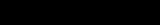xCantor setOverview

In mathematics
Mathematics
Mathematics is the study of quantity, space, structure, and change. Mathematicians seek out patterns and formulate new conjectures. Mathematicians resolve the truth or falsity of conjectures by mathematical proofs, which are arguments sufficient to convince other mathematicians of their validity...

, the Cantor set is a set of points lying on a single line segment
Line segment
In geometry, a line segment is a part of a line that is bounded by two end points, and contains every point on the line between its end points. Examples of line segments include the sides of a triangle or square. More generally, when the end points are both vertices of a polygon, the line segment...

that has a number of remarkable and deep properties. It was discovered in 1875 by Henry John Stephen Smith
Henry John Stephen Smith
Henry John Stephen Smith was a mathematician remembered for his work in elementary divisors, quadratic forms, and Smith–Minkowski–Siegel mass formula in number theory...

and introduced by German
Germany
Germany , officially the Federal Republic of Germany , is a federal parliamentary republic in Europe. The country consists of 16 states while the capital and largest city is Berlin. Germany covers an area of 357,021 km2 and has a largely temperate seasonal climate...

mathematician
Mathematician
A mathematician is a person whose primary area of study is the field of mathematics. Mathematicians are concerned with quantity, structure, space, and change....

Georg Cantor
Georg Cantor
Georg Ferdinand Ludwig Philipp Cantor was a German mathematician, best known as the inventor of set theory, which has become a fundamental theory in mathematics. Cantor established the importance of one-to-one correspondence between the members of two sets, defined infinite and well-ordered sets,...

in 1883.

Through consideration of it, Cantor and others helped lay the foundations of modern general topology
General topology
In mathematics, general topology or point-set topology is the branch of topology which studies properties of topological spaces and structures defined on them...

. Although Cantor himself defined the set in a general, abstract way, the most common modern construction is the Cantor ternary set, built by removing the middle thirds of a line segment.